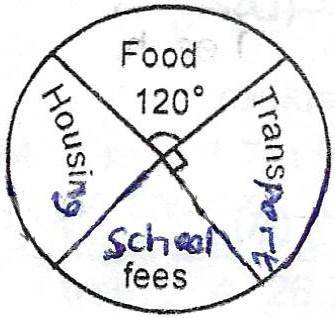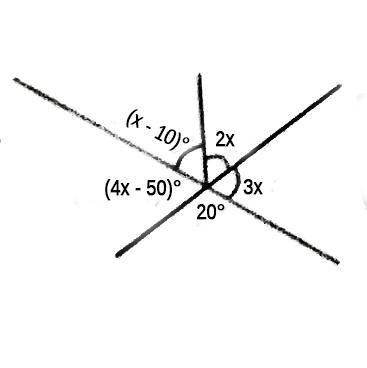## Mathematics 2018 Past Questions | JAMB

Study the following Mathematics past questions and answers for JAMBWAEC  NECO and Post-JAMB. Get prepared with official past questions and answers for upcoming examinations.

1. In a class of 40 students, 32 offer Mathematics, 24 offer Physics and 4 offer neither Mathematics nor Physics. How many offer both Mathematics and Physics?

• A. 4
• B. 8
• C. 16
• D. 20

2.  Find the values of x for which (x + 2) / 4 -(2x -3)/3 < 4

• A. x < 8
• B. x > -6
• C. x < 4
• D. x > -3

3.The pie chart shows the monthly expenditure of a public servant. The monthly expenditure on housing is twice that of school fees. How much does the worker spend on housing if his monthly income is N7200?

• A. 1000
• B. 2000
• C. 3000
• D. 4000

4.  A trader realises 10x – x$$^2$$ Naira profit from the sale of x bags of corn. How many bags will give him the maximum profit?

• A. 7
• B. 6
• C. 5
• D. 4

5.  If y = 23$$_{5}$$ + 101$$_{3}$$ , find y, leaving your answer in base two

• A. 1110
• B. 10111
• C. 11101
• D. 111100

6.Find the value of x in the diagram

• A. 10°
• B. 28°
• C. 36°
• D. 40°

7. Solve for t in the equation $$\frac{3}{4}$$t + $$\frac{1}{3}$$(21 – t) = 11

• A. $$\frac{9}{13}$$
• B. $$\frac{7}{13}$$
• C. 5
• D. 9$$\frac{3}{5}$$

8.  A school girl spends $$\frac{1}{4}$$ of her pocket money on books and $$\frac{1}{3}$$ on dress. What fraction remains?

• A. $$\frac{5}{6}$$
• B. $$\frac{7}{12}$$
• C. $$\frac{5}{12}$$
• D. $$\frac{1}{6}$$

9.  If $$\frac{x}{a + 1}$$ + $$\frac{y}{b}$$ = 1. Make y the subject of the relation.

• A. $$\frac{b(a + 1 – x)}{a + 1}$$
• B. $$\frac{a + 1}{b(a – x + 1)}$$
• C.$$\frac{a(b – x + 1)}{b + 1}$$
• D. $$\frac{b}{a(b – x + 1)}$$

10. Calculate the total surface area of a cupboard which measures 12cm by 10cm by 8cm

• A. 1920cm²
• B. 592cm²
• C. 296cm²
• D. 148cm²

## All Subjects

Subscribe
Notify ofInline Feedbacks